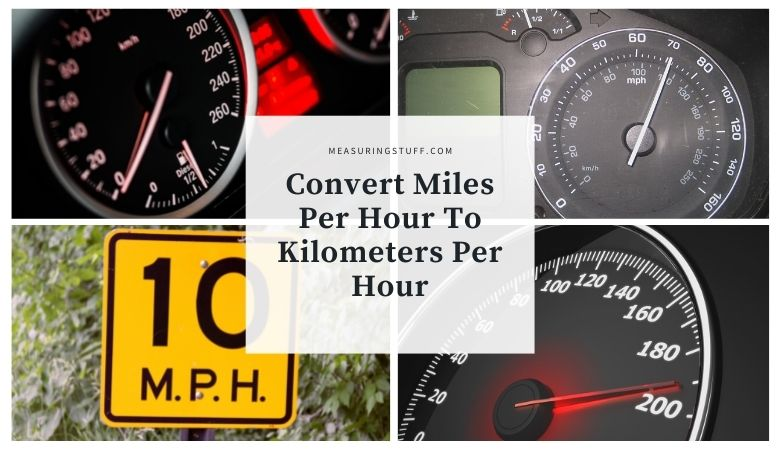# Convert Miles Per Hour To Kilometers Per Hour (MPH To KPH)

Last updated on March 20th, 2023 at 12:53 pm

Similar to converting kilometers per hour into miles per hour, this can be done easily by using the following formula.

There are 1.609344 kilometers per hour in 1 mile per hour.

Simply multiply any miles per hour by 1.609344 to convert into kilometers per hour.

For example, 1 MPH x 1.609344 = 1.609344 KPH.

Another example:

Convert 120 MPH into KPH.

120 x 1.609344 = 193.121 KPH.

Some people will round off the multiple to 1.6 for easier math without a calculator handy.

Converting kilometers per hour into miles per hour can be done by multiplying the KPH by 0.62137119223733.

For example, 1 KPH x 0.62137119223733 = 0.62137119223733 MPH.

Another example:

Convert 120 KPH into MPH.

120 x 0.62137119223733 = 74.564 MPH.

You can also refer to the following table to convert MPH into KPH and vice versa.

### Miles per hour to kilometers per hour conversion calculator

Use the following calculator to easily convert MPH into KPH.

## How many MPH is 100 KPH?

100 kilometers per hour equals 62.137119223733 miles per hour.

CHECK OUT  How Much Weight Can A Golf Cart Hold?

Multiply 100 by 0.62137119223733 to convert KPH into MPH.

100 x 0.62137119223733 = 62.137119223733

## How many KPH is 100 MPH?

100 miles per hour equals 160.9344 kilometers per hour.

Multiply 100 by 1.609344 to convert MPH into KPH.

100 x 1.609344 = 160.9344